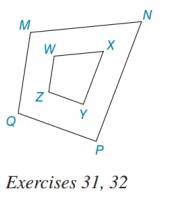Chapter 5.1, Problem 32EElementary Geometry For College St...

7th Edition
Alexander + 2 others
ISBN: 9781337614085

Solutions

Chapter
SectionElementary Geometry For College St...

7th Edition
Alexander + 2 others
ISBN: 9781337614085
Textbook Problem

For this exercise, use the drawing and extended ratio of Exercise 31. If N P = 2 ⋅ X Y and WZ = 3 1 2 ., to find MQ.To determine

To find:

The value of MQ.

Explanation

According to Means-Extremes Property, in a proportion, the product of the means equals the product of the extremes.

Calculation:

It is given that MNWX=NPXY=PQYZ=MQWZ, NP=2XY and WZ = 312=72.

Consider, NPXY=MQWZ

Substitute NP=2XY and WZ = 312 in NPXY=MQWZ

Still sussing out bartleby?

Check out a sample textbook solution.

See a sample solution

The Solution to Your Study Problems

Bartleby provides explanations to thousands of textbook problems written by our experts, many with advanced degrees!

Get Started

Find more solutions based on key concepts